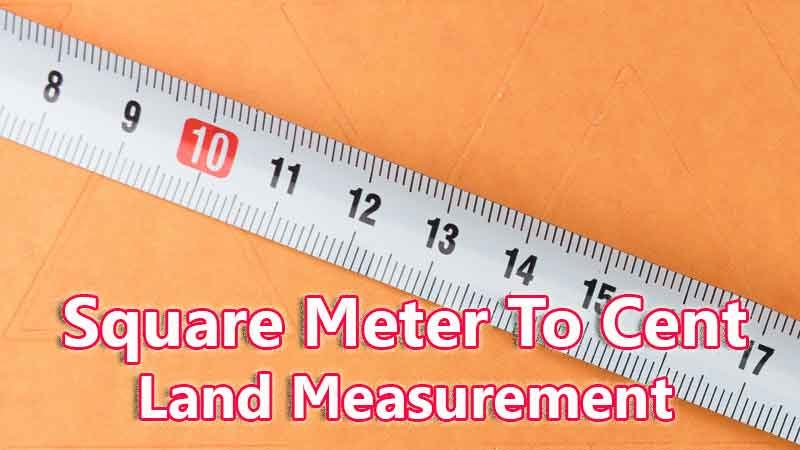# Square Meter To Cent Converter

Square Meter is a traditional land unit used to measure the land Area standardly. Here we are using the square meter to cent unit value conversion. And square meter also comes under these land area units like Gonda, Kani, and cent. In West Bengal, One square meter is directly equivalent to 0.02471 cent.

Input Here

square meter

Output

cent

1 square meter = 0.02471 cent

## Measurement

square meter means - a unit of land measurement. This tool provides great calculation over square meter to cent, 1 square meter to cent, 1 square meter in cent, 1 square meter equal to and etc. To convert square meter to cent, then multiply the unit value by 0.02471. Then, 1 square meter * 0.02471 = 0.02471 cent. Hence, 1 square meter is equal to 0.02471 cent.

cent also comes under this land measurement unit and we are comparing values between square meter traditional unit with other side land measurement unit.

## Formula For square meter to cent Conversion(square meter to cent formula)

The base formula for this square meter to cent converter is

cent = square meter * 0.02471

## How many cent in a square meter?

Step 1: To Convert 1 square meter to cent

Step 2: Applying formula cent = square meter * 0.02471, (i.e) multiply the unit value by 0.02471.

Step 3: Then, cent = 1 * 0.02471 = 0.02471.

Step 4: Hence, 1 square meter is equal to 0.02471 cent.Some square meter to cent Conversion Chart for your reference:

 1 square meter = 0.02471 cent 2 square meter = 0.04943 cent 3 square meter = 0.07414 cent 4 square meter = 0.09885 cent 5 square meter = 0.12356 cent 6 square meter = 0.14828 cent 7 square meter = 0.17299 cent 8 square meter = 0.1977 cent 9 square meter = 0.22242 cent 10 square meter = 0.24713 cent

The square meter to cent conversion chart is above listed for your reference. This chart, however, represents the simple math calculation involved in the square meter to cent convertion online.

For Example: How much is 1 square meter to cent

Solution:

= (square meter * 0.02471)

= (1 x 0.02471)

= 0.02471 cent

For Example: How much is 120 square meter in cent

Solution:

= (square meter * 0.02471)

= (120 x 0.02471)

= 2.96554 cent

This formula provides an instant answer for all your questions / People also search:

• 1 square meter to cent
• 1 square meter is equal to how many cent
• Formula to convert square meter to cent online
• How to convert square meter to cent?
• How much cent is equal to a one square meter?
• How many cent in square meter?
• 1 square meter equal to cent